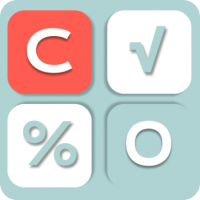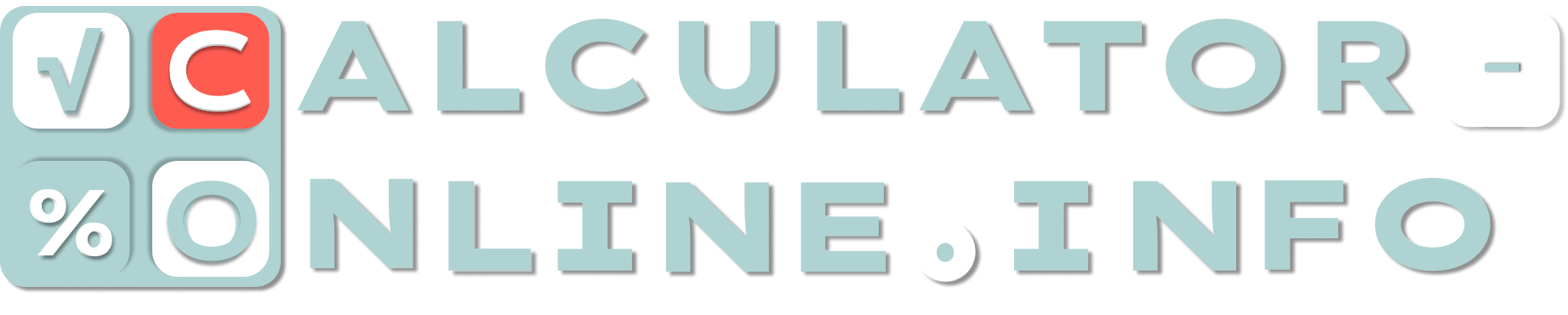# Age Calculator

How old am I calculator is the service that consist of two tools. The first one calculates how old you are for now, while the second count the age due to any date you want.

## Age calculator to current day

Calculate how old you are today by the date of birth

## Age calculator to exact day

Calculate how old you were or you will be at a certain point in time
What date you are interested in:

## How old are you?

To determine your age in year it is enough to subtract the smaller year (the year you were born) from the larger one. For example, if you were born in 1998, you need to subtract it from the year you are interested in. Let it be 2023, so that we could subtract 1998 from 2023. The result will be 25 years. How accurate is this result? Since we do not take into account the month and date, it is not entirely accurate, because your birthday can be either at the beginning of the year, or in the second half, or even at the end.

It means that usual age calculator by year is good but not accurate. Instead of it, we offer our calculators:

1. Age calculator by date of birth that will calculate your exact age for today.
2. Age calculator by birthdate that will calculate your exact age for any date.

Both age calculators will take into account not only years, but also months with days, so you will know the exact result.

## Birthday age calculator — how it works

Age calculator calculates the time span from birthday up to the current date or any another date. You only need to indicate the date and day of your birth. This is enough to determine the time until the current day, but if you need to calculate the time interval from the birthday to another day, it is enough to specify it using the second calculator.

As a result, by birthday age calculator can count the exact age of the user in years, months and days.

Rating
( 2 assessment, average 5 from 5 )Mathematician/ author of the article